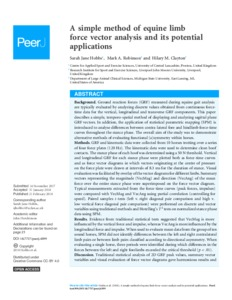# A simple method of equine limb force vector analysis and its potential applications.

Hobbs, SJ, Robinson, MA and Clayton, HM (2018) A simple method of equine limb force vector analysis and its potential applications. PeerJ, 6. ISSN 2167-8359Preview
Text
2018 Hobbs PeerJ Equine GRF.pdf - Published Version
Available under License Creative Commons Attribution.

Download (8MB) | Preview

## Abstract

Background: Ground reaction forces (GRF) measured during equine gait analysis are typically evaluated by analyzing discrete values obtained from continuous force-time data for the vertical, longitudinal and transverse GRF components. This paper describes a simple, temporo-spatial method of displaying and analyzing sagittal plane GRF vectors. In addition, the application of statistical parametric mapping (SPM) is introduced to analyse differences between contra-lateral fore and hindlimb force-time curves throughout the stance phase. The overall aim of the study was to demonstrate alternative methods of evaluating functional (a)symmetry within horses. Methods: GRF and kinematic data were collected from 10 horses trotting over a series of four force plates (120 Hz). The kinematic data were used to determine clean hoof contacts. The stance phase of each hoof was determined using a 50 N threshold. Vertical and longitudinal GRF for each stance phase were plotted both as force-time curves and as force vector diagrams in which vectors originating at the centre of pressure on the force plate were drawn at intervals of 8.3 ms for the duration of stance. Visual evaluation was facilitated by overlay of the vector diagrams for different limbs. Summary vectors representing the magnitude (VecMag) and direction (VecAng) of the mean force over the entire stance phase were superimposed on the force vector diagram. Typical measurements extracted from the force-time curves (peak forces, impulses) were compared with VecMag and VecAng using partial correlation (controlling for speed). Paired samplest-tests (left v. right diagonal pair comparison and high v. low vertical force diagonal pair comparison) were performed on discrete and vector variables using traditional methods and Hotelling'sT2tests on normalized stance phase data using SPM. Results: Evidence from traditional statistical tests suggested that VecMag is more influenced by the vertical force and impulse, whereas VecAng is more influenced by the longitudinal force and impulse. When used to evaluate mean data from the group of ten sound horses, SPM did not identify differences between the left and right contralateral limb pairs or between limb pairs classified according to directional asymmetry. When evaluating a single horse, three periods were identified during which differences in the forces between the left and right forelimbs exceeded the critical threshold (p < .01). Discussion: Traditional statistical analysis of 2D GRF peak values, summary vector variables and visual evaluation of force vector diagrams gave harmonious results and both methods identified the same inter-limb asymmetries. As alpha was more tightly controlled using SPM, significance was only found in the individual horse althoughT2plots followed the same trends as discrete analysis for the group. Conclusions: The techniques of force vector analysis and SPM hold promise for investigations of sidedness and asymmetry in horses.

Item Type: Article Equine; Force; GRF; Gait analysis; Lameness; Locomotion; Vector diagram Q Science > QL ZoologyQ Science > QP Physiology Sport & Exercise Sciences PeerJ Author 16 Mar 2018 10:55 20 Sep 2018 01:47 10.7717/peerj.4399 http://researchonline.ljmu.ac.uk/id/eprint/8319

### Actions (login required)View Item